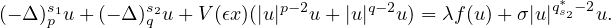Electron. J. Differential Equations, Vol. 2021 (2021), No. 100, pp. 1-24.

### Existence results for nonlinear Schrodinger equations involving the fractional (p,q)-Laplacian and critical nonlinearities Huilin Lv, Shenzhou Zheng, Zhaosheng Feng

Abstract:
In this article, we consider the existence of ground state positive solutions for nonlinear Schrodinger equations of the fractional (p,q)-Laplacian with Rabinowitz potentials defined in Rn,We prove existence by confining different ranges of the parameter λ under the subcritical or critical nonlinearities caused by σ=0 or 1, respectively. In particular, a delicate calculation for the critical growth is provided so as to avoid the failure of a global Palais-Smale condition for the energy functional.

Submitted May 18, 2021. Published December 20, 2021.
Math Subject Classifications: 35R11, 35A15, 58E05.
Key Words: Nonlinear Schrodinger equations; nonlocal (p,q)-Laplacian; critical growth; Rabinowitz potentials; Nehari manifold.

Show me the PDF file (422 KB), TEX file for this article.Huilin Lv Department of Mathematics Beijing Jiaotong University Beijing 100044, China email: huilinlv@bjtu.edu.cn Shenzhou Zheng Department of Mathematics Beijing Jiaotong University Beijing 100044, China email: shzhzheng@bjtu.edu.cn Zhaosheng Feng School of Mathematical and Statistical Sciences University of Texas Rio Grande Valley Edinburg, TX 78539, USA email: zhaosheng.feng@utrgv.edu